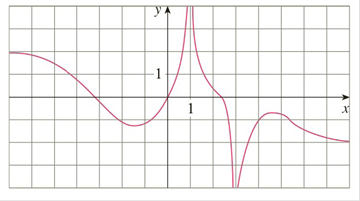Chapter 3.4, Problem 3E

Chapter
Section
Textbook Problem

# For the function f whose graph is given, state the following.(a) lim x → ∞ f ( x ) (b) lim x → − ∞ f ( x ) (c) lim x → 1 f ( x ) (d) lim x → 3 f ( x ) (e) The equations of the asymptotesTo determine

a)

To state:

limx∞ f(x) from the given graph.

Explanation

1) Concept:

Use the definition of limit at infinity.

2) Definition:

Limit at infinity:

Let f be function defined on (a,). Then

limxf(x)=L

Means that the values of f(x) can be made arbitrarily close to L by requiring x to be sufficiently large.(means, values of f(x) approaches L as x becomes larger and larger.)

3) Given:

limx∞ f(x)

4) Calculation:

From the given graph, as x, fx-2

limx∞ f(x)=-2

Conclusion:

Therefore,limx∞ f(x)=-2

b)

To state:

limx-∞ f(x) from the given graph.

Solution:

limx-∞ f(x)=2

1) Concept:

Use the definition of limit at infinity.

2) Definition:

Limit at infinity:

Let f be function defined on (a,). Then

limx-f(x)=L

Means that the values of f(x) can be made arbitrarily close to L by requiring x to be sufficiently large negative.(means, values of f(x) approaches L as x becomes larger and larger negative)

3) Given:

limx-∞ f(x)

4) Calculation:

From the given graph, as x-, fx2

limx-∞ f(x)=2

Conclusion:

Therefore, limx-∞ f(x)=2

c)

To state:

limx1 f(x) from given graph

Solution:

limx1 f(x)=

1) Concept:

Use the definition of infinite limit [From chapter 1, (1.6 Definition 4)]

2) Intuitive definition of an infinite limit:

Let f be a function defined on both sides of a, except possibly at a itself. Then

limxf(x)=

Means that the values of f(x) can be made arbitrarily large by taking x sufficiently close to a, but not equal to a

3) Given:

limx1 f(x)

4) Calculation:

### Still sussing out bartleby?

Check out a sample textbook solution.

See a sample solution

#### The Solution to Your Study Problems

Bartleby provides explanations to thousands of textbook problems written by our experts, many with advanced degrees!

Get Started